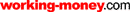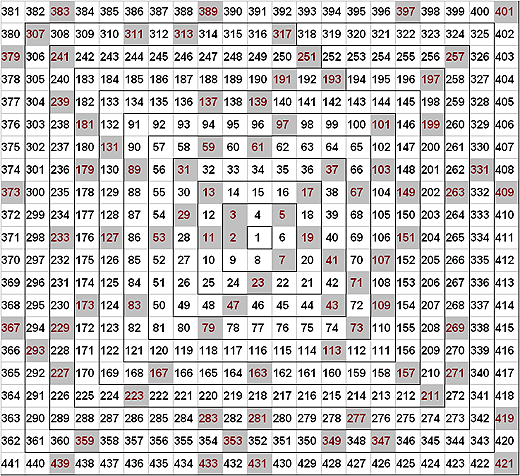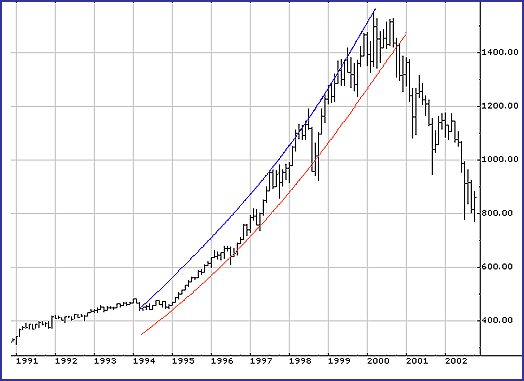HOT TOPICS LIST

LIST OF TOPICS

# Gann's Square Of Nine

12/18/03 09:10:16 AM PST
by Gary Goldberg

Whether you call it Gann's square of nine or something else, it holds secrets to the greatest mystery in mathematics -- the distribution of prime numbers.

If ever there was a bit of trading mumbo-jumbo that I counted as not worth thinking about, it was W.D. Gann's square of nine. How could I bother with century-old jargon about prices vibrating off each other, square-outs of time, prices related to degrees of a circle, producing a mystical numerology that purports to give exact dates on which particular prices must be reached?

Nonetheless, I decided to make another attempt to understand it when Jeff Cooper, a trader whom I (otherwise) respect, described his use of the Gann square in a RealMoney.com article. Something in the article jogged my memory, and it was then that I realized Gann's square of nine is in fact known to mathematicians in a context totally unrelated to the stock market. To mathematicians, the square of nine is known as Ulam's Prime Number Spiral, and displays some of the properties of the greatest unsolved mystery in mathematics the distribution of the prime numbers.

### SIGNIFICANCE OF PRIME NUMBERS

What could these two fields trading the stock market and prime number theory possibly have in common? Puzzled, I could not find a writer, trader or mathematician, who had mentioned the equivalence of the spirals of Ulam and Gann; Jeff Cooper confirmed to me that he had never heard of this connection. If it has been noticed before, it is at least not well known. Is it possible there is some hidden relationship here that could profit a trader? Could prime numbers be turned into prime cash? I decided to track down the connection.

Gann's square of nine consists of the integers arranged in the form of a square spiral. One can construct it using a spreadsheet or graph paper. Place the number 1 in a central cell; to its left place the number 2; above that is 3, and move around clockwise, adding one each time: to the right of 3 is 4 and 5, then below 5 is 6 and then 7. To the left of 7 is 8 and then 9, which completes the first square. To the left of 9 place 10 to start a new square out, and above 10 place 11, and so on, around and around as large as one likes (Figure 1). Gann believed this arrangement captured a hidden law that the markets inexorably followed.Figure 1: The Gann square of nine. The mathematician Stanislaw Ulam discovered that the prime numbers shown in blue sometimes fall unexpectedly along lines in the square, such as in the diagonal ray extending from the center to the northeast corner.

In trading, the numbers are interpreted as prices, and their circular arrangement represents the passage of time; a full turn around the spiral takes one to the next larger square and represents a single period, such as a year. Of particular significance are lines of numbers that fall along the eight cardinal directions of a compass: the four rays emanating from the center in the directions of north, east, south and west identify the alignment of prices that occur at certain times of the year, as do the set of diagonals running from the center to northwest, northeast, southwest and southeast.

Gann traders look for prices at significant highs and lows then check along these significant rays to find other prices at which future highs and lows are expected to occur. Traders can also find price relationships by looking at angles of 90 degrees, 180 degrees, and so forth, that are formed with respect to a significant price. For example, in the first square, 9 is related to 3 by a 90-degree turn, and 7 is related to 3 by 180 degrees. With these tools, Gann traders make forecasts of the size of price oscillations and the timing of significant turning points in the markets. Gann himself apparently used these methods to predict wheat prices in 1909 that established his fame.

The figure known to mathematicians as "Ulam's prime number spiral" is constructed in exactly the same fashion as the square of nine, but its use is quite different. It was discovered by mathematician Stanislaw Ulam in 1963. While listening to a "long and very boring paper" at a science conference, Ulam drew a square grid, thinking he might set up a chess problem; instead, he began numbering the intersections of the grid by spiraling out from the center. Then he idly began to circle the prime numbers the only integers that cannot be calculated by multiplying together other integers. He was astounded to find that sets of prime numbers arranged themselves along diagonal lines in the diagram. See Figure 1, where the primes are in the shaded cells; note the sequences such as 7, 23, 47, and 79, or 5, 19, 41, 71, 109.

Subsequently programming a Maniac II computer to generate a picture of the distribution confirmed that prime numbers appeared along diagonal lines throughout the largest square spirals that could be made. For example, Gann's 45-degree line, the northeast ray, holds the primes 5, 17, 37, 101, 197, 257, 401, and so on: 70% of the numbers out to the 10th square are primes, even though only about 20% of the integers up to 401 are prime.

Prime numbers are important because they act as the "elementary particles" of the numbers: all integers can be uniquely calculated by multiplying together only primes. The distribution of the prime numbers among the other integers is considered by many to be the greatest unsolved mathematical mystery.

The patterns that leapt to the eye in Ulam's spiral indicate some underlying order to the distribution of the primes that has yet to be fully understood. Some people regard the mystery of the prime numbers with an awe that rivals Gann's mystical search for the hidden laws of the stock market. But what brings these two mysteries together into the square spiral?

### NATURAL SQUARES CALCULATOR

The answer to this question, although only a partial one, is that Gann's square of nine is a parabola calculator. Gann referred to it as the "natural squares calculator" and may have derived it from ancient techniques for computing squares of numbers. Note that the natural squares 22 = 4, 32 = 9, 52 = 25 and so on fall along the southwest diagonal of the square. In fact, each of the cardinal rays extending from the center is described by a quadratic equation, which on a time-price chart forms a parabolic curve.

Let us label the ever-larger concentric squares with integers n. The center square, with just the number 1, we will label as n = 0; the next square out, with three unit cells on a side and largest number 9, will be labeled n =1, the next, with five cells on a side and largest number 25, is n = 2, and so on, so that the nth square has sides of length 2n+1 cells.

The area of the nth square is then (2n+1) 2 = 4n2 + 4n + 1, and this is also the largest number in each square (equal to the number of cells in the square). These largest numbers per square are lined up along the ray extending from the center to the southwest. Thus, the southwest ray corresponds to the quadratic expression 4n2 + 4n + 1.

All eight rays important to Gann theory are described by quadratic expressions of the form 4n2 + an + 1, where the choice of the coefficient a determines the angle of the ray. Gann called the ray pointing to the east the "zero degree ray," and so each of the others correspond to successive increments of 45 degrees; using this identification, the coefficient a that generates the parabola corresponding to the ray at angle w is given by a = 1 - w/45, where w takes on the values between 180 degrees and minus 180 degrees.

On a stock chart of price versus time, formulas of the form 4n2 + an + 1 produce a parabola, where the parameter n represents time and the expression evaluates to the corresponding price. Gann's contention that prices along the same ray "vibrate" against each other is actually a way of saying that sometimes critical prices fall along a parabola, which is a fact of trading life: blowoff tops occur when price goes parabolic. Thrusts increase in length and pullbacks decrease; parabolas can also describe the slowing down and turning of a trend as thrusts become smaller and pullbacks greater.

When Gann describes price moving between rays of different angles he is describing the motion of the stock price between members of a fanlike family of parabolas, effectively describing a parabolic trend channel. To good approximation, all linear trend channels eventually become parabolic trend channels as markets turn. Gann's square of nine and his use of esoteric language disguise genuine phenomena that traders can find useful.

What does this have to do with primes? In Ulam's spiral, the curious alignments of prime numbers is also related to the spiral's function as a parabola calculator. In the 18th century, mathematicians Legendre and Euler discovered that some sequences of primes can be generated by quadratic expressions. A particularly famous expression is x2 + x + 41, published by Euler in 1772, which has 40 consecutive primes. These prime-generating quadratic polynomials appear as lines in the square of nine.

Although most of these lines do not pass through the center, with suitable labeling of the squares they can be described by parabolas of the form 4n2 + an + b. In the case of the sequence of primes 5, 19, 41, 71, the parabola has coefficients a = 10 and b = 5, and primes are generated for n = 0, 1, 2, 3, as well as various higher n. For the sequence 7, 23, 47, 79 the corresponding coefficients are a = 7 and b = -1 for n starting at 1.

Not every number along these lines is a prime, but an unusually high proportion are. According to the prime number theorem, the chance that any randomly selected integer x is a prime is roughly one over the natural log of x; the frequency of primes along some of the lines in the Ulam spiral far exceed that random distribution.

Ulam and his coworkers showed that along some diagonals, almost half the numbers less than 10,000,000 are prime. Just why this is so is still a mystery of mathematics. There are partial explanations. For example; some diagonals across the spiral can contain no primes on them because their quadratic expressions can be factored into the product of two linear expressions, and as a consequence there must be clusters of primes elsewhere; but it's not clear this explains the consistency of the distribution.

A Gann square of nine in which any given line of primes passes through the center and falls along the Gann cardinal directions can be formed by suitable adjustment of the central and incremental numbers that generate the square. By starting with -1 in the center of the square rather than 1, the 5, 19, 41. . . sequence becomes the southeast ray while the 7, 23, 47. . . sequence becomes the southwest ray.

Adjusting the center element can generate other prime rays; placing 17 or 41 in the center square generates spirals in which every number along a diagonal is a prime out to the 16th and 40th squares, respectively. These rays correspond to two of the most famous prime-generating quadratic expressions.

A Gann trader with an eye for primes could look for market price oscillations among prime-number parabolic channels. For example, in Figure 2, the Standard & Poor's 500 from 1994 to 2000 was supported by the parabola that corresponds to the northeast ray of the standard square of nine, which happens to be the ray with the most prime numbers of the cardinal directions. The upper curve of the parabolic channel corresponded to the ray 180 degrees opposite, the southwest ray, which happens to have absolutely no primes.Figure 2: A Gann parabolic channel for the S&P 500 from 1994 to 2000. The upper parabola corresponds to the southwest ray in the square of nine, starting at the 10th square; the lower parabola is the northeast ray, starting at the ninth square. Time is scaled so that one complete turn of the square is equal to nine months.

Personally, I find it difficult to believe that prime numbers can be particularly useful in trading unless they have a psychological effect: traders of any methodology might instinctively see prime numbers as special or offbeat (as they do not appear as results in multiplication tables learned in school), and thus provide a more attractive place for entry and exit stops than the more obvious numbers ending in zero or the simple fractions of 10.

A better lesson to be drawn is that both Ulam and Gann were able to use this unusual arrangement of numbers to visually detect patterns that otherwise might be difficult to find. This is a technique that any seeker of hidden relationships can put to use. Whatever its meaning, I can't help but believe Gann would have been pleased to find his square of nine vibrating with a deeply hidden order of mathematics.

Gary Goldberg is a physicist and software developer. He trades from Silicon Valley.

Gardner, Martin . "The Remarkable Lore Of The Prime Numbers," Scientific American 210 (March), reprinted in The Sixth Book Of Mathematical Games From Scientific American (Freeman, 1971).

Stein, M.L., S.M. Ulam, and M.B. Wells . "A Visual Display Of Some Properties Of The Distribution Of Primes," American Mathematical Monthly 71 (May).

### ON THE WEB

Cooper, Jeff . "An Introduction to the Wheel of Price and Time," RealMoney.com, October 9, www.thestreet.com/p/rmoney/jeffcooper/10046607.html

Peterson, Ivar . "Prime Spirals," Science News Online, May 4, www.sciencenews.org/20020504/mathtrek.asp

Wolfram Research, "Prime Spiral," mathworld.wolfram.com/PrimeSpiral.html

"W.D. Gann's Square of Nine," Lambert-Gann Publishing Co., www.wdgann.com/TradingArticles/articles.htm

"Square of Nine Generator," www.xmlworks.com/gann/

"Primality Test," Prime Pages, primes.utm.edu/curios/includes/file.php?file=primetest.html

Current and past articles from Working Money, The Investors' Magazine, can be found at Working-Money.com.

Gary Goldberg

 Comments or Questions? Article Usefulness 5 (most useful) 4 3 2 1 (least useful)